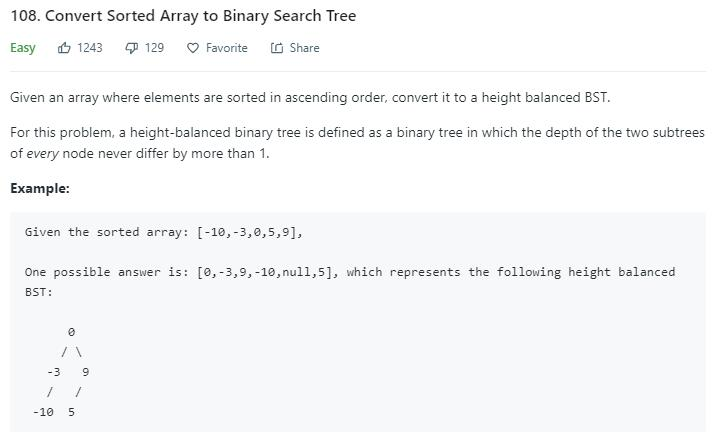# 题目描述（简单难度）1. 若任意节点的左子树不空，则左子树上所有节点的值均小于它的根节点的值；
2. 若任意节点的右子树不空，则右子树上所有节点的值均大于它的根节点的值；
3. 任意节点的左、右子树也分别为二叉查找树；
4. 没有键值相等的节点。

# 解法一 递归

public TreeNode sortedArrayToBST(int[] nums) {
return sortedArrayToBST(nums, 0, nums.length);
}

private TreeNode sortedArrayToBST(int[] nums, int start, int end) {
if (start == end) {
return null;
}
int mid = (start + end) >>> 1;
TreeNode root = new TreeNode(nums[mid]);
root.left = sortedArrayToBST(nums, start, mid);
root.right = sortedArrayToBST(nums, mid + 1, end);

return root;
}


# 解法二 栈 DFS

class MyTreeNode {
TreeNode root;
int start;
int end
MyTreeNode(TreeNode r, int s, int e) {
this.root = r;
this.start = s;
this.end = e;
}
}


Stack<MyTreeNode> rootStack = new Stack<>();
int start = 0;
int end = nums.length;
int mid = (start + end) >>> 1;
TreeNode root = new TreeNode(nums[mid]);
TreeNode curRoot = root;
rootStack.push(new MyTreeNode(root, start, end));


while (end - start > 1) {
mid = (start + end) >>> 1; //当前根节点
end = mid;//左子树的结尾
mid = (start + end) >>> 1;//左子树的中点
curRoot.left = new TreeNode(nums[mid]);
curRoot = curRoot.left;
rootStack.push(new MyTreeNode(curRoot, start, end));
}


MyTreeNode myNode = rootStack.pop();
//当前作为根节点的 start end 以及 mid
start = myNode.start;
end = myNode.end;
mid = (start + end) >>> 1;
start = mid + 1; //右子树的 start
curRoot = myNode.root; //当前根节点
if (start < end) { //判断当前范围内是否有数
mid = (start + end) >>> 1; //右子树的 mid
curRoot.right = new TreeNode(nums[mid]);
curRoot = curRoot.right;
rootStack.push(new MyTreeNode(curRoot, start, end));
}


class MyTreeNode {
TreeNode root;
int start;
int end;

MyTreeNode(TreeNode r, int s, int e) {
this.root = r;
this.start = s;
this.end = e;
}
}
public TreeNode sortedArrayToBST(int[] nums) {
if (nums.length == 0) {
return null;
}
Stack<MyTreeNode> rootStack = new Stack<>();
int start = 0;
int end = nums.length;
int mid = (start + end) >>> 1;
TreeNode root = new TreeNode(nums[mid]);
TreeNode curRoot = root;
rootStack.push(new MyTreeNode(root, start, end));
while (end - start > 1 || !rootStack.isEmpty()) {
//考虑左子树
while (end - start > 1) {
mid = (start + end) >>> 1; //当前根节点
end = mid;//左子树的结尾
mid = (start + end) >>> 1;//左子树的中点
curRoot.left = new TreeNode(nums[mid]);
curRoot = curRoot.left;
rootStack.push(new MyTreeNode(curRoot, start, end));
}
//出栈考虑右子树
MyTreeNode myNode = rootStack.pop();
//当前作为根节点的 start end 以及 mid
start = myNode.start;
end = myNode.end;
mid = (start + end) >>> 1;
start = mid + 1; //右子树的 start
curRoot = myNode.root; //当前根节点
if (start < end) { //判断当前范围内是否有数
mid = (start + end) >>> 1; //右子树的 mid
curRoot.right = new TreeNode(nums[mid]);
curRoot = curRoot.right;
rootStack.push(new MyTreeNode(curRoot, start, end));
}

}

return root;
}


# 解法三 队列 BFS

class MyTreeNode {
TreeNode root;
int start;
int end;

MyTreeNode(TreeNode r, int s, int e) {
this.root = r;
this.start = s;
this.end = e;
}
}
public TreeNode sortedArrayToBST3(int[] nums) {
if (nums.length == 0) {
return null;
}
TreeNode root = new TreeNode(0);
rootQueue.offer(new MyTreeNode(root, 0, nums.length));
while (!rootQueue.isEmpty()) {
MyTreeNode myRoot = rootQueue.poll();
int start = myRoot.start;
int end = myRoot.end;
int mid = (start + end) >>> 1;
TreeNode curRoot = myRoot.root;
curRoot.val = nums[mid];
if (start < mid) {
curRoot.left = new TreeNode(0);
rootQueue.offer(new MyTreeNode(curRoot.left, start, mid));
}
if (mid + 1 < end) {
curRoot.right = new TreeNode(0);
rootQueue.offer(new MyTreeNode(curRoot.right, mid + 1, end));
}
}

return root;
}


# 扩展 求中点

int mid = (start + end) / 2


(start + end) / 2 = (start + end + start - start) / 2 = start + (end - start) / 2


int mid = (start + end) >>> 1


>>有符号右移就会出现问题了，事实上 JDK6 之前都用的>>，这个 BUG 在 java 里竟然隐藏了十年之久。